##### TI-Nspire For Dummies, 2nd EditionThe Solve, Factor, and Expand commands form the cornerstone of the Algebra submenu. However, several other items contained on the Algebra submenu are worth mentioning.

In the first screen, the Solve a System of Equations tool is accessed, which automatically starts a wizard. Use this tool to solve systems of equations. Technically, the wizard correctly places the syntax for the Solve command.

You could have accessed the Solve command directly, but why try to remember the syntax if you can use a wizard? The second line of the second screen shows how the solution to a dependent system is displayed. The infinite number of solutions is indicated by an expression in terms of the constant c2. This constant can equal any real number and gives the set of ordered pairs that lie on the line 2xy = –3.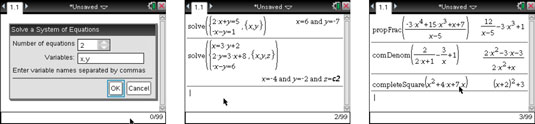To open the Proper Fraction tool press [MENU]→Algebra→Fraction Tools→Proper Fraction. This tool is very useful for doing long division of polynomials or finding the slant asymptote of a rational function. Another fraction tool (only available on a TI-Nspire CAS) is the Common Denominator tool. The newest tool in the Algebra submenu, the Complete the Square tool, puts a quadratic equation into vertex form for a parabola.

Following is a list of some of the other functions found on the Algebra submenu and a brief description of what they do:

• Zeros: The syntax for this command is Zeros(Expression, Variable). This command produces a list of the values of the specified variable that make the expression equal zero.

Sometimes the Zero command returns the empty set because the zeros are complex. To find complex zeros, press [MENU]→Algebra→Complex→Zeros.

• Polynomial tools: This submenu contains the following polynomial commands:

• Remainder of Polynomial: The syntax for this command is polyRemainder(Expression1, Expression2) and returns the remainder when Expression1 is divided by Expression2.

• Quotient of Polynomial: The syntax for this command is polyQuotient(Expression1, Expression2) and returns the quotient when Expression1 is divided by Expression2, less the remainder.

• Greatest Common Divisor: The syntax for this command is polyGcd(Expression1, Expression2) and returns the greatest common rational factor of Expression1 and Expression2.

• Coefficients of Polynomial: The syntax for this command is polyCoeffs(Expression) and returns the numerical coefficients, in list form, of the polynomial Expression. Coefficients are given in order from the highest degree term down to the lowest degree term. For example, polyCoeffs(5x + x3 – 3) returns the list {1, 0, 5, –3}. Notice that a zero is given for the missing x2 term.

• Degree of Polynomial: The syntax for this command is polyDegree(Expression) and returns the degree of the polynomial.

• Convert Expression tools: This submenu contains the following commands:

• Convert to ln: The syntax for this command is Expression ¢ln and returns an expression only containing natural logs.

• Convert to logbase: The syntax for this command is Expression1 ¢logbase(Expression2) and returns a simplified expression using base Expression2.

• Convert to exp: The syntax for this command is Expression ¢exp and returns an expression in terms of the natural exponential, e.

• Convert to sin: The syntax for this command is Expression ¢sin and returns an expression in terms of sine.

• Convert to cos: The syntax for this command is Expression ¢cos and returns an expression in terms of cosine.

• Trigonometry: This submenu contains the following trigonometry commands:

• Expand: The syntax for this command is tExpand(Expression) and returns the expansion of sines and cosines whose angles are integer multiples, sums, and differences. For example, the expression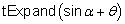returns the angle-sum identity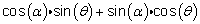This is a great command to use if you forget the sum, difference, double, and half-angle trigonometric formulas.

• Collect: The syntax for this command is tCollect(Expression) and returns an expression in which powers and products of sines and cosines are converted to linear combinations of sines and cosines of multiple angles, angle sums, and angle differences. Basically, the tCollect command reverses the results obtained by the tExpand command. For example, the expression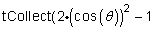gives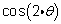as a result.

Greek characters can be found in the Symbol palette. The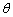character can also be found by pressing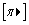and choosing